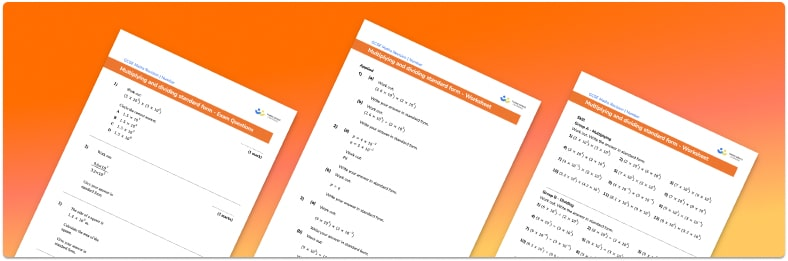# Multiplying And Dividing Standard Form Worksheet• Section 1 of the multiplying and dividing standard form worksheet contains 36 skills-based multiplying and dividing standard form questions, in 3 groups to support differentiation
• Section 2 contains 3 applied multiplying and dividing standard form questions with a mix of worded problems and deeper problem solving questions
• Section 3 contains 4 foundation and higher level GCSE exam style multiplying and dividing standard form questions
• Answers and a mark scheme for all multiplying and dividing standard form questions are provided
• Questions follow variation theory with plenty of opportunities for students to work independently at their own level
• All questions created by fully qualified expert secondary maths teachers
• Suitable for GCSE maths revision for AQA, OCR and Edexcel exam boards

• This field is for validation purposes and should be left unchanged.

You can unsubscribe at any time (each email we send will contain an easy way to unsubscribe). To find out more about how we use your data, see our privacy policy.

### Multiplying and dividing standard form at a glance

Standard form, or standard index form, is a simple way of writing very large numbers or very small numbers. To write numbers in standard form, we write them decimals between 1 and 10, multiplied by powers of 10.  We call numbers that are not written in standard form ordinary numbers.

We can multiply and divide numbers in standard form directly by multiplying or dividing the decimals and then multiplying or dividing the powers of 10. For example, (4×102)x(2×105)=4x2x102x105. 4×2=8 and simplifying the powers of 10 gives us 107.  Therefore (4×103)x(2×105)=8×107.

After multiplying or dividing the decimal, we may need to adjust the position of the decimal place to ensure the answer is written in standard form. To do this we need to make sure the decimal in the answer is still between 1 and 10 by adjusting the power of 10 to maintain the place value of the number.

Alternatively we can convert the numbers in standard form to ordinary numbers, multiply them together and then convert the answer back to standard form. When converting ordinary numbers to standard form we start by writing any significant figures as a decimal between 1 and 10. We then need to maintain the place value of the number by multiplying the decimal by a power of 10. For example, the number 2500 can be written as 2.5×103. For very small numbers, the powers of ten will be negative powers.

Looking forward, students can then progress to additional number worksheets, for example aor aFor more teaching and learning support on Number our GCSE maths lessons provide step by step support for all GCSE maths concepts.

## Do you have KS4 students who need more focused attention to succeed at GCSE?There will be students in your class who require individual attention to help them succeed in their maths GCSEs. In a class of 30, it’s not always easy to provide.

Help your students feel confident with exam-style questions and the strategies they’ll need to answer them correctly with our dedicated GCSE maths revision programme.

Lessons are selected to provide support where each student needs it most, and specially-trained GCSE maths tutors adapt the pitch and pace of each lesson. This ensures a personalised revision programme that raises grades and boosts confidence.

Find out more